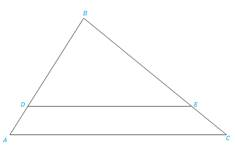Chapter 5.3, Problem 25E### Elementary Geometry for College St...

6th Edition
Daniel C. Alexander + 1 other
ISBN: 9781285195698

#### Solutions

Chapter
Section### Elementary Geometry for College St...

6th Edition
Daniel C. Alexander + 1 other
ISBN: 9781285195698
Textbook Problem
6 views

# In Exercises 25 to 28, Δ A B C ∼ Δ D B EExercises 25-28Given AC = 8, DE = 6, CB = 6.Find: EB(HINT: Let EB = x, and solve an equation.)

To determine

To find:

The side EB when the given sides are AC = 8, DE = 6, CB = 6 and ΔABCΔDBE.

Explanation

Definition:

CSSTP:

Corresponding sides of similar triangles are proportional.

Description:

The given figure is shown below.

Figure 1

From the given figure, it is observed that there are two triangles ABC and DBE and which is given that two triangles are similar.

Now draw the two triangles separately as shown below.

Figure 2

Figure 3

Since ΔABCΔDBE and from the definition of CSSTP, corresponding sides of similar triangles are proportional.

That is, BDBA=BEBC=DEAC.

It is given that AC = 8, DE = 6, CB = 6.

Find the side EB.

Consider the last two pairs of proportions BEBC=DEAC in order to find EB

### Still sussing out bartleby?

Check out a sample textbook solution.

See a sample solution

#### The Solution to Your Study Problems

Bartleby provides explanations to thousands of textbook problems written by our experts, many with advanced degrees!

Get Started

#### Find more solutions based on key concepts